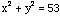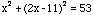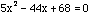Date: Sun, 28 Dec 1997 11:55:49 PST

Name: Sandy
Who is asking the question: teacher

Qustion:
The sum of the squares of two numbers is 53. Twice the greater, minus the lesser is 11. Find the numbers.

Hi Sandy

Suppose that the numbers are x and y with x>y. Your two conditions then areand
2x - y = 11.

Solve the second condition for y to get y = 2x - 11 and then substitute into the first givingwhich, when simplified, is. Solving, using the general quadratic, gives x=2 or x=6.8. Since y = 2x - 11 the two numbers are 2 and -7 or 6.8 and 2.6.

Cheers,
Penny.

Go to Math Central

To return to the previous page use your browser's back button.